A Quadrilateral in which a pair of opposite sides have the same length and are inclined at 60° to each other (or equivalently, satisfy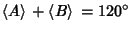). Some interesting theorems hold for such quadrilaterals. Let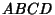be an equilic quadrilateral with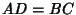and. Then

1. The Midpoints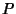,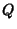, and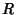of the diagonals and the side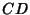always determine an Equilateral Triangle.

2. If Equilateral Triangle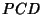is drawn outwardly on, then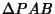is also an Equilateral Triangle.

3. If Equilateral Triangles are drawn on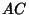,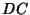, and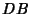away from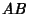, then the three new Vertices,, andare Collinear.

See Honsberger (1985) for additional theorems.

References

Garfunkel, J. The Equilic Quadrilateral.'' Pi Mu Epsilon J. 7, 317-329, 1981.

Honsberger, R. Mathematical Gems III. Washington, DC: Math. Assoc. Amer., pp. 32-35, 1985.